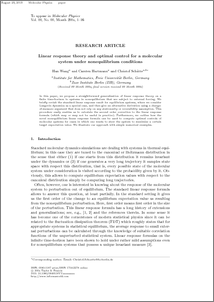Repository: Freie Universität Berlin, Math Department

# Linear response theory and optimal control for a molecular system under nonequilibrium conditions

Wang, H. and Hartmann, C. and Schütte, Ch. (2013) Linear response theory and optimal control for a molecular system under nonequilibrium conditions. Molecular Physics, 111 . pp. 3555-3564. ISSN 0026-8976Preview
PDF - Submitted Version
413kB

Official URL: http://dx.doi.org/10.1080/00268976.2013.844370

## Abstract

In this paper, we propose a straightforward generalization of linear response theory to systems in nonequilibrium that are subject to nonequilibrium driving. We briefly revisit the standard linear response result for equilibrium systems, where we consider Langevin dynamics as a special case, and then give an alternative derivation using a change-of-measure argument that does not rely on any stationarity or reversibility assumption. This procedure moreover easily enables us to calculate the second order correction to the linear response formula (which may or may not be useful in practice). Furthermore, we outline how the novel nonequilibirum linear response formula can be used to compute optimal controls of molecular systems for cases in which one wants to steer the system to maximize a certain target expectation value. We illustrate our approach with simple numerical examples.

Item Type: Article Mathematical and Computer Sciences > Mathematics > Applied Mathematics Department of Mathematics and Computer Science > Institute of MathematicsOther Institutes > Matheon > A - Life SciencesDepartment of Mathematics and Computer Science > Institute of Mathematics > Cellular Mechanics GroupDepartment of Mathematics and Computer Science > Institute of Mathematics > BioComputing Group 1297 Carsten Hartmann 01 Jul 2013 13:05 03 Mar 2017 14:41

Repository Staff Only: item control page